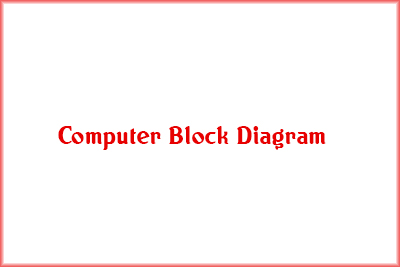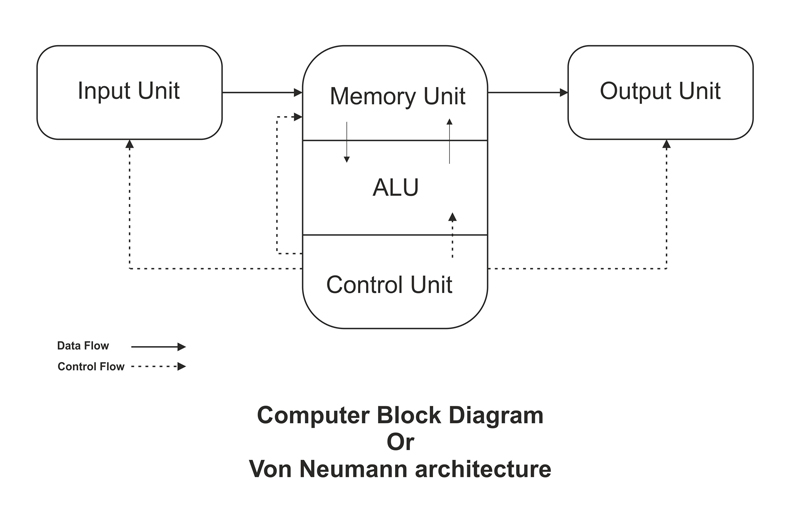Block Diagram of Computers
24-07-2019    293 timesJohn von Neumann (1903 -1957) was a Hungarian-American mathematician, physicist and computer scientist design first EDVAC diagram in 1945. Later it was famous as neue von Architecture or block diagram of computer system. Still today we follow the same diagram. It has three parts

• Input device
• Central Processing Unit
• Output Device1. Input Devices: These devices are used to input raw data into the computer. The raw data are a set of letters, numbers, images etc.
• Keyboard
• Mouse
• Scanner
• Barcode Scanner
• Joystick
• WebCam
2. Central Processing Unit: The memory unit, control unit and ALU of the computer are together known as the Central Processing Unit (CPU). The CPU is known as brain of the computer. It performs the following functions:
• All calculations.
• All decisions.
• Controls all units of the computer.

A PC may have a CPU-IC such as Intel 8088, 80286, 80386, 80486, Celeron, Pentium, Pentium Pro, Pentium II, Pentium III, Pentium IV, Dual Core, and AMD Core 2 duo, quad Core etc.

1. Memory Unit: Two types of internal memory are (i) Register Memory and (ii) Cache Memory
1. Register memory: Register memories are storing all the temporary data of the process which run on the CPU. It is maximum 16 bit memory.
2. Cache Memory: This is the fastest memory in the computer which is used to synchronize the speed of data transfer when it is transferring data from any low speed device to high speed device. Cache memories generally used to transfer data from primary memory (RAM) to register memory and vice versa.
2. Arithmetic Logical Unit: All calculations made by a computer are actually done by the Arithmetic Logic Unit (ALU). It also performs the logical calculation and takes decisions. This part of CPU performs basic arithmetic operation like +, - , /, *, % etc. and logical operation like >, <, = … etc. all the required data for calculation is coming from memory unit and after performing the calculation it send to the output device through the memory unit. If the output device is busy then the result store or spooling in the memory unit.
3. Control Unit: The main function of the controlled unit of the computer is control all other parts of that computer. It guides the input device to receive the data from the user and also where and how the data will store. After then it controls the data flow between memory unit and ALU. It also controls the data flow of the result from the ALU to memory unit and memory unit to the output device.

e.g. A Human can perform a simple sum like 19+34 =?, But if we provide 1,00,000 simple problems of the same type and give a time span of 5 min, then he or she will make a mistake (due to lack of concentration). But the computer does not make any mistake in this situation, due to the control unit.3. Output Unit: After processed the raw fact computer generate the information which is displayed or gives through the output unit of a computer. Output is also called as Result. We can also save these results in the storage devices for the future use. Some common output devices are
• Printers
• Visual Display Unit (VDU)
• Sound Box
• Projectors
• plotter